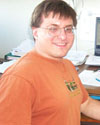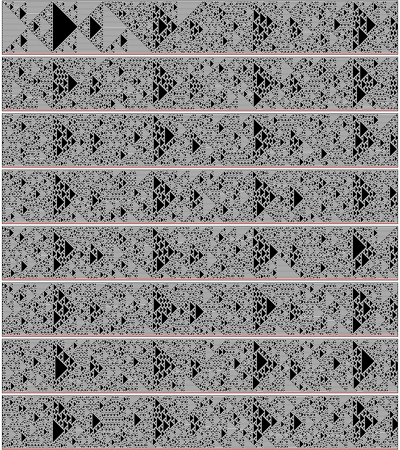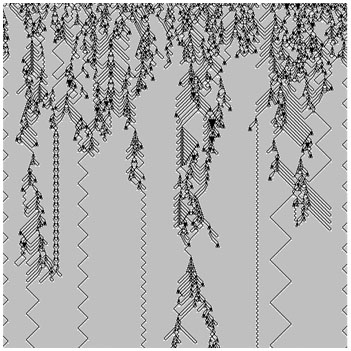#Wolfram Summer School

# Alumni# Konstantin Kouptsov

Summer School

Class of 2007

## Bio

My formal training is in mathematical/theoretical physics. I have been studying physics at the Moscow Engineering Physics Institute in Russia, and now hold a PhD degree from New York University. Currently, I am working as a Design Analyst at Wolfram Research Inc., where my job is to analyze, define and document functional designs for software and web products. My other interests are quite interdisciplinary and cover selected topics in computer science, mathematics, and biology.

## Project: Quasiperiodicity

A line in the configuration space misses periodic points by a small amount.

RepeatList[s_List,n_Integer]:=With[{r=Length[s]},With[{p=Mod[n,r],q=
Quotient[n,r]},Flatten[{Table[s,{q}],Take[s,p]}]]];
wall=Sequence[{2,x_,_}:>0,{_,2,_}-&gt;2,{_,x_,2}:>0];
With[{w=128,length=992},ev=CellularAutomaton[{rule,wall},
Join[{0,2,0,1},RepeatList[{0,0,1,1,0,0},w],{0,2,0}],8*length];
Column[ArrayPlot[Transpose[#],PixelConstrained-&gt;1,ColorRules-&gt;{0-&gt;
White,1-&gt;Black,2-&gt;Red}]&@Partition[ev,length]]]## Favorite Outer Totalistic Three-Color Rule

Rule chosen: 11828070This reflects various types of computations, which are pretty complex; but who knows what they are doing?

APPLY NOW